`What products are involved in this article?This article is based on the use of RCP (Rheonics Control Panel) connected to the SME (Smart Module Electronics) operating SRD, DVP, and DVM. Hence it can be used for any of these Rheonics sensors.`
`What is the purpose of this article?Explain the process to get a temperature compensated density when it has a linear trend. Usual applications are with fluids with a linear dependency on temperature.`

`1. Temperature-compensated Density`

Density, defined as the substance's mass per unit of volume is an important fluid property that has an increasing presence in monitoring processes and control systems.

Density is affected by temperature and pressure. For most liquids, the Density decreases with temperature. As pressure increases, the density of any stable material also increases, markedly in gases, and almost negligibly in liquids and solids.

In most cases, we want to monitor the fluid density at a constant reference temperature. If the process temperature isn’t constant, an additional source of error to the control system is added. To avoid the temperature dependence on the fluid’s density and truly monitor its consistency, the temperature compensated density is introduced.

To compensate for temperature effects, the Rheonics Control Panel offers the following models Linear, Polynomial, or ASTM functions.

`2. Math model`

The SRD sensor can measure inline density, viscosity, and temperature. With the use of mathematical models, temperature compensated measurements can be obtained.

Below is the mathematical formula for the density linear model used in the RCP (Rheonics software).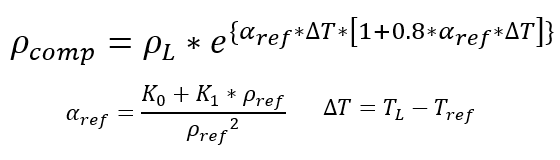Equation 1: Density ASTM Model.

Where:

ρcomp - Compensated density, calculated by the Sensor.

ρL - Line density, read by the Sensor.

ρref - Reference density, is the density around which the Sensor will compensate the readings. This is defined and should be specified by the user.

K0 and K1 - Coefficients, specified by user.

TL - Temperature, read by the Sensor.

Tref - Reference temperature, is the temperature around which the Sensor will compensate the readings. This is defined and should be specified by the user.

`3. Checking the data`

The first step to achieving a compensated density requires the user to take samples of density at different temperatures, these temperatures must be higher and lower than the normal operation temperature range in your process. Once enough data is obtained, the user should define the reference temperature. For this example, the blue point (15°C) is used as the typical set-point temperature of the process line.

The following steps outline the correct procedure for data analysis:

3.1.  Select the reference temperature and density from data obtained in tests. Here, reference temperature and density are highlighted in blue in the next Table, we want to create a correlation at 15°C where we expect a density of  917 Kg/m3.

 Temperature [°C] Density [Kg/m3] 9 923 10 920.4 15 917 20 913.7 25 910.4 29 907.7

Table 1. Data points from inline measurements.

3.2. Plot data points of Denisty vs Temperature to study the behavior of the data.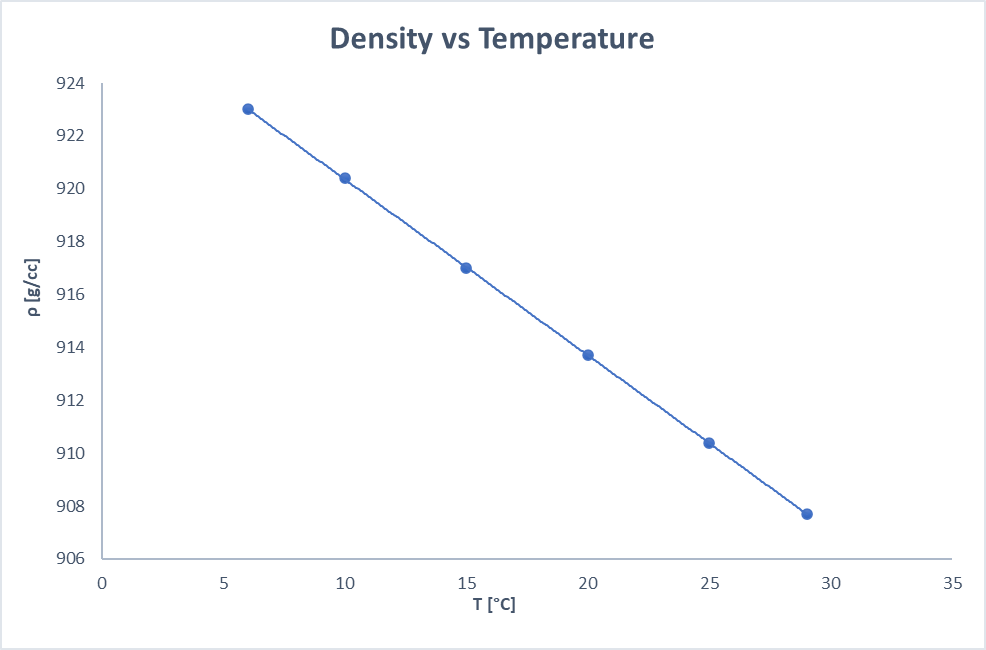Figure 1: Plot chart Density vs Temperature

3.3. The K1 and K0 values can be found in the API-ASTM-IP petroleum measurement tables, as shown below.

 Temperature [°C] Density [g/cc] K0 K1 Crude Oil 771 - 981 613.97226 0.00000 Gasolines 654 - 779 346.42278 0.43884 Kerosines 779 - 839 594.54180 0.00000 Fuel Oils 839 - 1075 186.96960 0.48618

Table 2. API-ASTM-IP petroleum measurement table

Using Crude Oil in this example, their values are as follows:

K1=613.97 and K0=0

Considering that:

Tref = 15°C

With all the input parameters set on the Sensor through the RCP software, the temperature compensated density will look like the next graph in Figure 2.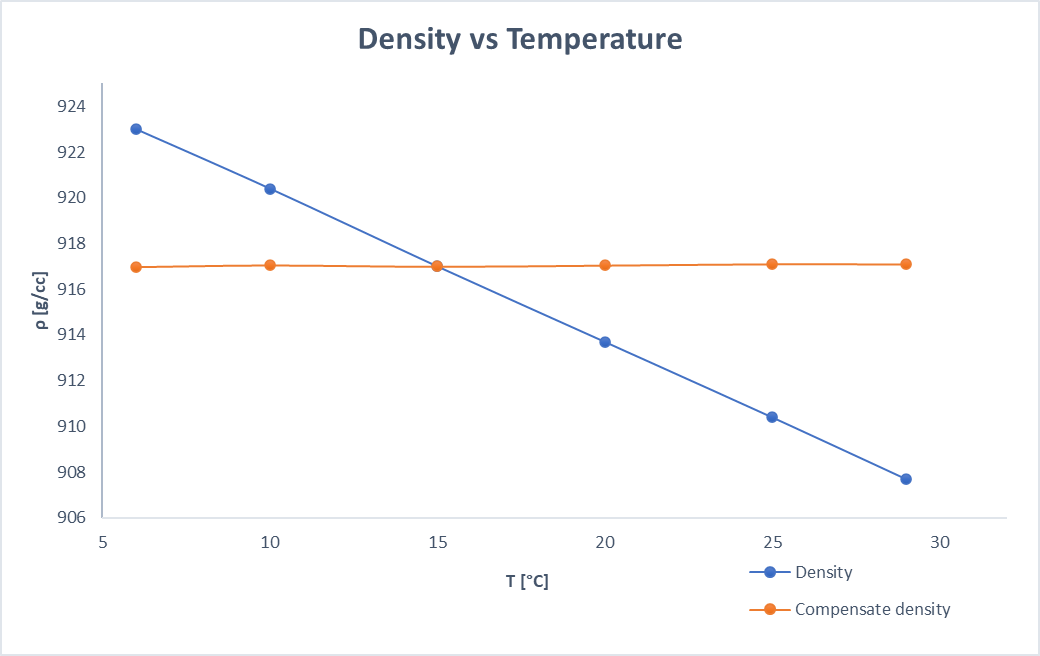Figure 2. Expected results when applying the mathematical model.

`4. How to load models into the Sensor electronics?`

The calculation tab on RCP allows configuring the Sensor to run mathematical models for viscosity, density, and concentration. Expert mode needs to be enabled for this.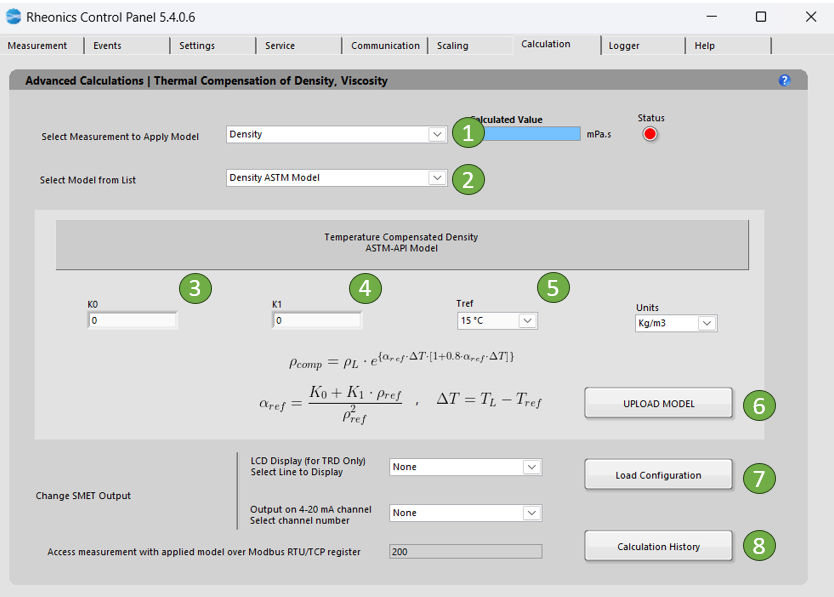Figure 3. Calculation tab for the exponential model of density.

The following steps are needed to load the models into the Sensor electronics:

1. In the dropdown list, “Select Measurement to Apply Model” density should be as default parameter.  If not, select it from the options in the list.

2. In “Select Model from list” select the model you would like to use for the specific parameter. When you select the model, you will get more information about the specific equation used and the coefficients you will need to apply to that model.  Select “Density ASTM model”

3. Input “K0” coefficient. For this example, it has a value of 613.97.

4. Input “K1” coefficient. For this example, it has a value of 0,0000.

5. Input reference temperature is used to find the coefficients models. In this example is 15°C.

6. Click “Upload Model” to load the model settings into Sensor electronics. The button should turn GREEN for a couple of seconds if the action was successful or turn RED if the operation was unsuccessful. This action will refresh the values for the uncompensated and compensated indicators. Here you can verify if the model is calculating the expected values. If not, you can correct and repeat the process.

7. Click “Load Configuration” to update the display and channel configurations in Sensor electronics.

4.1. Handling Sensor Outputs

For the SME-TRD, the compensated viscosity values can be shown on the display. Select the line to display the parameter: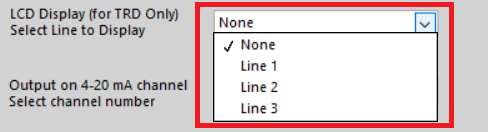Figure 4. Selecting read line for LCD display.

Select the analog channel to send data, this can be verified in the “Service” Tab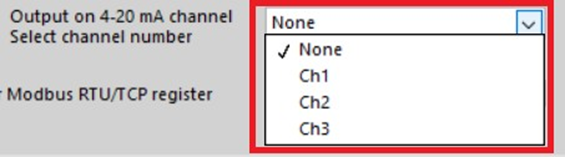Figure 5. Selecting analog output channel

Measurement can always be accessed at the register address that is displayed.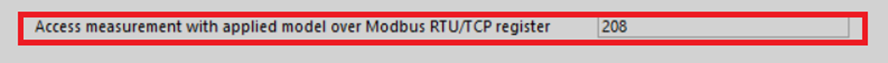Figure 6. Modbus TCP/IP register

4.2 Demonstration Video

Video 1. Configuration Density ASTM model in RPC Software.